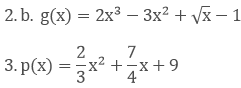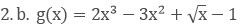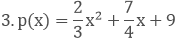×#### Thank you for registering.

One of our academic counsellors will contact you within 1 working day.

Click to Chat

1800-1023-196

+91-120-4616500

CART 0

• 0

MY CART (5)

Use Coupon: CART20 and get 20% off on all online Study Material

ITEM
DETAILS
MRP
DISCOUNT
FINAL PRICE
Total Price: Rs.

There are no items in this cart.
Continue Shopping• Complete JEE Main/Advanced Course and Test Series
• OFFERED PRICE: Rs. 15,900
• View Details

```Chapter 6: Factorization of Polynomials Exercise – 6.1

Question: 1

Which of the following expressions are polynomials in one variable and which are not?

1. 3x2 − 4x + 15

2. y2 + 2√3

3. 3√x + √2 x

4. x − 4/x

5. x12 + y2 + t50

Solution:

1. 3x2 − 4x + 15 it is a polynomial of x

2. y2 + 2√3 it is a polynomial of y

3√x + √2 x it is not a polynomial since the exponent of 3√x is not a positive term

4. x − 4/x- it is not a polynomial since the exponent of - 4/x is not a positive term

5. x12 + y2 + t50 it is a three variable polynomial which variables of x, y, t

Question: 2

Write the coefficients of x2 in each of the following

1. 17 − 2x + 7x2

2. 9 − 12x + x24. √3 x – 7

Solution:

Given, to find the coefficients of x2

1. 17 − 2x + 7x2 - the coefficient is 7

2. 9 − 12x + x2 - the coefficient is 0- the coefficient is π/6

4. √3 x - 7 - the coefficient is 0

Question: 3

Write the degrees of each of the following polynomials:

1. 7x3 + 4x2 − 3x + 12

2. 12 − x + 2x2

3.  5y - √2

4. 7 − 7x°

5. 0

Solution:

Given, to find degrees of the polynomials

Degree is highest power in the polynomial

1. 7x3 + 4x2 − 3x + 12 the degree is 3

2. 12 − x + 2x2 the degree is 3

3. 5y - √2 the degree is 1

4. 7 − 7x° the degree is 0

5. 0 the degree of 0 is not defined

Question: 4

1. x + x2 + 4

2. 3x - 2

3. 2x + x2

4. 3y

5. t2 + 1

f. 7t4 + 4t2 + 3t − 2

Solution:

Given

1. x + x2 + 4 - it is a quadratic polynomial as its degree is 2

2. 3x – 2 - it is a linear polynomial as its degree is 1

3. 2x + x2 - it is a quadratic polynomial as its degree is 2

4. 3y - it is a linear polynomial as its degree is 1

5. t2 + 1- it is a quadratic polynomial as its degree is 2

f. 7t4 + 4t2 + 3t – 2 - it is a bi-quadratic polynomial as its degree is 4

Question: 5

Classify the following polynomials as polynomials in one variables, two - variables etc:

1. x2 − xy + 7y2

2. x2 − 2tx + 7t2 − x + t

3. t3 − 3t2 + 4t − 5

4. xy + yz + zx

Solution:

Given

1. x2 − xy + 7y2 it is a polynomial in two variables x and y

2. x2 − 2tx + 7t2 − x + t it is a polynomial in two variables x and t

3. t3 − 3t2 + 4t − 5 it is a polynomial in one variable t

4. xy + yz + zx it is a polynomial in 3 variables in x , y and z

Question: 6

Identify the polynomials in the following:

1. f(x) = 4x3 − x2 − 3x + 74. q(x) = 2x2 − 3x + 4/x + 2

5. h(x) = x4 − x3/2 + x − 1

6. f(x) = 2 + 3x + 4x

Solution:

Given

1. f(x) = 4x3 − x2 − 3x + 7 it is a polynomialit is not a polynomial since the exponent of √x is a negative integerit is a polynomial as it has positive integers as exponents

4. q(x) = 2x2 − 3x + 4/x + 2 it is not a polynomial since the exponent of 4/x is a negative integer

5. h(x) = x4 − x3/2 + x − 1 it is not a polynomial since the exponent of - x3/2 is a negative integer

6. f(x) = 2 + 3x + 4x it is not a polynomial since the exponent of 3/x is a negative integer

Question: 7

Identify constant, linear, quadratic abd cubic polynomial from the following polynomials :

1. f(x) = 0

2. g(x) = 2x3 − 7x + 4

3. h(x) = −3x + 1/2

4. p(x) = 2x2 − x + 4

5. q(x) = 4x + 3

6. r(x) = 3x3 + 4x2 + 5x − 7

Solution:

Given,

1. f(x) = 0 as 0 is constant , it is a constant variable

2. g(x) = 2x3 − 7x + 4 since the degree is 3, it is a cubic polynomial

3. h(x) = −3x + 1/2 since the degree is 1, it is a linear polynomial

4. p(x) = 2x2 − x + 4 since the degree is 2, it is a quadratic polynomial

5. q(x) = 4x + 3 since the degree is 1, it is a linear polynomial

6. r(x) = 3x3 + 4x2 + 5x − 7 since the degree is 3, it is a cubic polynomial

Question: 8

Give one example each of a binomial of degree 25, and of a monomial of degree 100

Solution:

Given, to write the examples for binomial and monomial with the given degrees

Example of a binomial with degree 25 - 7x35 − 5

Example of a monomial with degree 100 - 2t100
```### Course Features

• 728 Video Lectures
• Revision Notes
• Previous Year Papers
• Mind Map
• Study Planner
• NCERT Solutions
• Discussion Forum
• Test paper with Video Solution